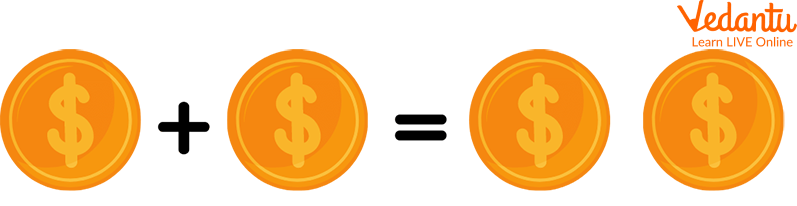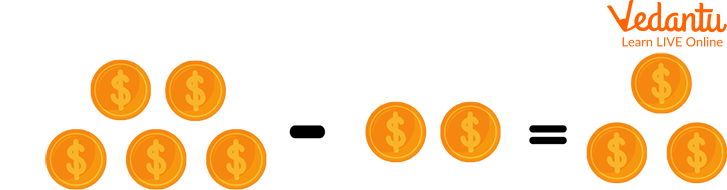Courses
Courses for Kids
Free study material
Offline Centres
More

# Addition and Subtraction of MoneyLast updated date: 03rd Dec 2023
Total views: 99.6k
Views today: 2.99k## Introduction to Addition and Subtraction of Money

Money is the physical quantity through which we buy and sell things in our daily life. When we buy some items we need to pay the cost of that item in terms of money. And to be very perfect in buying and selling items we need to understand very well the concept of addition and subtraction of money. Whenever we buy multiple items from any shop we need to pay the amount which will be calculated by adding money but there is a possibility that we may not have an exact amount so we must also know how to subtract to be sure that the shopkeeper has not cheated us.

## Understanding the Concept of Money

In India, we very often refer to the rupee as money but that’s a wrong concept because anything that we use in order to exchange items or use can be termed as money. And “Rupee” is the currency that is used in our country to exchange items of our use. Different countries have different currencies to buy and sell items. In ancient times people used different things to exchange goods with one another such as grains in exchange for pottery and many more things.

Suppose we go to a vegetable shop and buy a kilogram(kg) of potato Rs.30 per kg, a kg of onion Rs.45 per kg, and a kg of ladyfinger Rs.20 per kg. Now while paying the amount to the shopkeeper what we need to do is that we need to add all the amounts and then give them to the shopkeeper. This is where we require the addition of money.

We also need to know a fact about the Indian Rupee when we add 1 paise, 100 times then we get a rupee.## Subtraction of Money

Take the above example of the vegetable shop after the shopkeeper tells you the total amount which is Rs.95, but you have a hundred rupee note with you which you give it to the shopkeeper. After that shopkeeper returns you Rs.5. What has just happened is the shopkeeper has subtracted the total cost of vegetables you purchased from the money that you gave to him and returned the remaining amount of money to you. This is useful in calculating the amount left with us after subtracting the expenses.Subtraction of Money

## Solved Examples

Below are some solved examples based on addition of rupees and paise along with the subtraction.

Q 1. Trisha went to a retail shop and bought the listed items:

 Items Quantity Cost Amount Rice 4kg Rs.40 /kg Rs.160 Dal 2kg Rs.60 /kg Rs.120 Maggi 3pkt. Rs.80 /kg Rs.240 Sugar 2kg Rs.40 /kg Rs.80 Chips 2pkt Rs.20 /kg Rs.40

What is the total amount of money that Trisha will pay in the retail shop? If she paid a thousand rupee note then what is the amount of money that the retailer will return?

Ans: To get the total amount of money that Trisha will pay we need to add the total cost of the product.

Now, the total cost of items = Rs. 160 + Rs. 120 + Rs. 240 + Rs. 80 + Rs. 40

= Rs. 640

Hence, the amount of money that Trisha will have to pay is Rs.640.

The amount which the retailer will return is = Rs. 1000 - Rs. 640

= Rs. 360

So, the amount returned was Rs. 360.

Q 2. Mr.Salim went to purchase a refrigerator worth Rs.22,768 and he also purchased a set of bottles. He paid Rs.23,000 to the shopkeeper. Calculate the cost of the bottle set.

Ans: The cost of the bottle set can be calculated by subtracting the cost of the refrigerator from the total amount paid by Mr.Salim.

Cost of bottle set = Rs. 23,000 - Rs. 22,768

= Rs. 232

So, the cost of the above bottle set is Rs.232.

## Practice Problems

Q 1. Calculate the cost of 12 cups if the cost of a cup is Rs.45 along with 6 spoons with the cost of Rs.12 per spoon.

Ans: Rs.612

Q 2. The cost of the gift purchased by Seeta is Rs.345 and she paid Rs.500 note to the shopkeeper. Calculate the amount returned to Seeta by the shopkeeper.

Ans: Rs.155

Q 3. The cost of flowers per piece is Rs.6 and Himi bought 12 flowers. Find the amount that she will have to pay to the shopkeeper.

Ans: Rs.72

## Summary

We have learned about the physical exchange of goods in terms of money. And we have learned why we need to know how to add and subtract money in our daily life. We have also come across some problems which actually occur in real life and how to solve those problems. The importance of addition and subtraction is actually experienced when we go to buy things from the market. We can visualize the practical example for this topic there. Hope that this would have made your concept clear.

## FAQs on Addition and Subtraction of Money

1. Why do we need to learn the addition and subtraction of money?

In addition to buying and selling we have to calculate the profit and loss in the transaction process and keep a record of our expenses and earnings so that we can plan our upcoming expenses and cater to our savings.

2. Is it possible to give a technical brief for money?

In technical language, money is the medium for exchange but it could be in the form of anything digital bonds, assets, or cash for which exchange could take place.

3. Which type of coins were used in ancient times for buying and selling?

In ancient times various types of coins were used which were made up of gold, silver, glass, copper, etc. because at that time gold was not so rare and kings were known by the amount of gold they had with them. In the 19th and 20th centuries, the paisa was used as a currency.